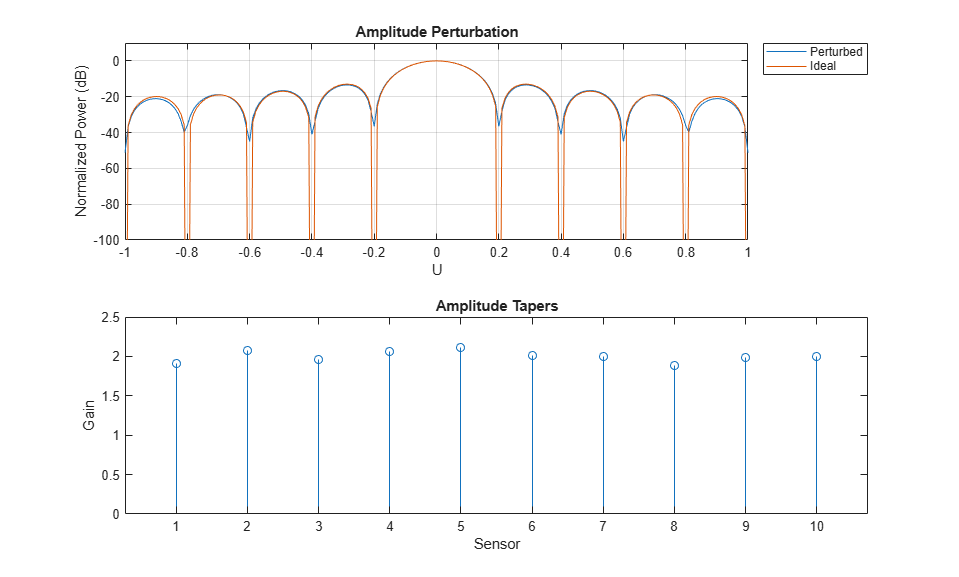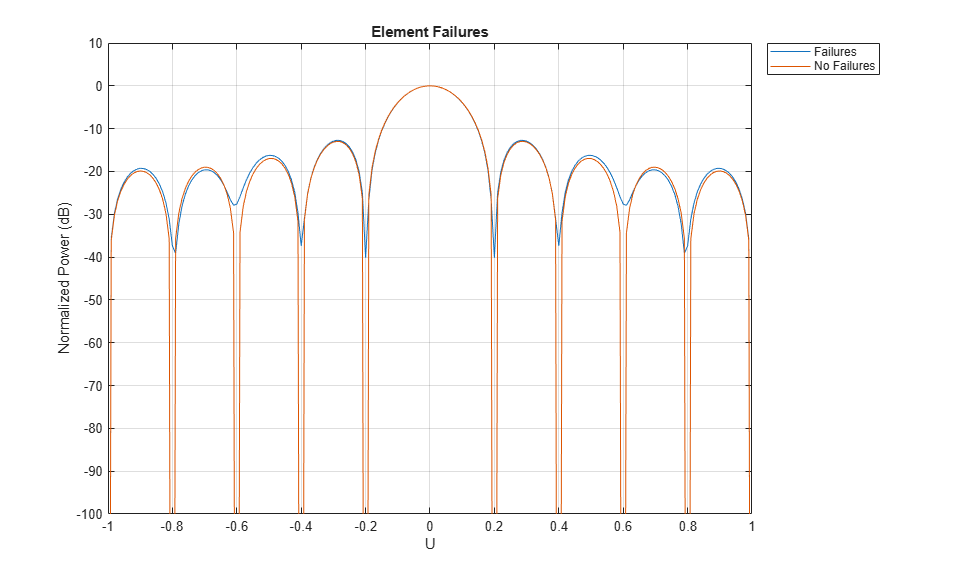# Modeling Perturbations and Element Failures in a Sensor Array

This example shows how to model amplitude, phase, position and pattern perturbations as well as element failures in a sensor array.

### Amplitude Perturbation

This section shows how to add gain or amplitude perturbations on a uniform linear array (ULA) of 10 elements. Consider the perturbations to be statistically independent zero-mean Gaussian random variables with standard deviation of 0.1.

Create a ULA antenna of 10 elements.

```N = 10; ula = phased.ULA(N); ```

Create amplitude or gain perturbations on the array

```rs = rng(7); pertStDev = 0.1; perturbations(ula,'TaperMagnitude','Normal',1,pertStDev); perturbedULA = perturbedArray(ula); ```

Compare the response of the perturbed to the ideal array.

```% Overlay responses c = 3e8; freq = c; subplot(2,1,1); helperCompareResponses(perturbedULA,ula,'Amplitude Perturbation', ... {'Perturbed','Ideal'}); % Show the corresponding tapers subplot(2,1,2); stem(perturbedULA.Taper) title('Amplitude Tapers');xlabel('Sensor');ylabel('Gain'); ```### Phase Perturbation

This section shows how to add phase perturbations to the ULA antenna used in the previous section. Consider the perturbations distribution to be similar to the previous section.

```perturbations(ula,'TaperMagnitude','none'); perturbations(ula,'TaperPhase','Normal',0,pertStDev); perturbedULA = perturbedArray(ula); ```

Compare the response of the perturbed to the ideal array.

```% Overlay responses subplot(2,1,1); helperCompareResponses(perturbedULA,ula,'Phase Perturbation', ... {'Perturbed','Ideal'}); % Show the corresponding tapers subplot(2,1,2); stem(angle(perturbedULA.Taper)) title('Phase Tapers');xlabel('Sensor');ylabel('Phase (rad)'); ```Notice how the perturbed response has shallower nulls.

### Position Perturbation

This section shows how to perturb the positions of the ULA sensor along the three axes.

```perturbations(ula,'TaperPhase','none'); perturbations(ula,'ElementPosition','Normal',0,pertStDev); perturbedULA = perturbedArray(ula); ```

Compare the response of the perturbed to the ideal array.

```% Overlay responses clf; helperCompareResponses(perturbedULA,phased.ULA(N), ... 'Position Perturbation', {'Perturbed','Ideal'}); ```View the array.

```viewArray(perturbedULA); title('Element Positions'); ```### Pattern Perturbation

This section will replace the isotropic antenna elements with perturbed patterns.

First create 10 custom antenna elements with perturbed isotropic patterns.

```antenna = phased.CustomAntennaElement; radpat = antenna.MagnitudePattern; element = cell(1,N); for i = 1:N perturbedAntenna = clone(antenna); perturbedAntenna.MagnitudePattern = ... pow2db(1+randn(size(radpat))*pertStDev); element{i} = perturbedAntenna; end ```

Here, map the 10 patterns in the cell array 'element' to the 10 sensors using the ElementIndices property.

```perturbedULA = phased.HeterogeneousULA('ElementSet',element, ... 'ElementIndices',1:N); ```

Compare the response of the perturbed to the ideal array.

```% Overlay responses clf; subplot(2,2,[1 2]); helperCompareResponses(perturbedULA,phased.ULA(N),... 'Pattern Perturbation', ... {'Perturbed','Ideal'}); % Show the perturbed pattern response next to the ideal isotropic pattern subplot(2,2,3); pattern(ula.Element,freq,'CoordinateSystem','polar','Type','power') title('Isotropic pattern'); subplot(2,2,4); pattern(element{1},freq,'CoordinateSystem','polar','Type','power') title('Perturbed pattern'); ```### Element Failures

This section will model element failures on an 8 by 10 uniformly rectangular array. Each element has 10 percent probability of failing.

Create a URA antenna of 8 by 10 elements.

```ura = phased.URA([8 10]); ```

Failures can be modeled by setting the gain on the corresponding sensor to 0. Here a matrix is created in which each element has a 10 percent probability of being 0.

```perturbations(ura,'ElementFailure','RandomFail',0.1); perturbedURA = perturbedArray(ura); % ura.Taper = double(rand(8,10) > .1); ```

Compare the response of the array with failed elements to the ideal array.

```% Overlay responses clf; helperCompareResponses(perturbedURA,ura,'Element Failures', ... {'Failures','No Failures'}); ```Notice how deep nulls are hard to attain in the response of the array with failed elements.

View the failed elements.

```viewArray(perturbedURA,'ShowTaper',true); title('Failed Elements'); % reset the random seed rng(rs) ```### Summary

This example showed how to model different kind of perturbations as well as element failures. It also demonstrated the effect on the array response for all the cases.